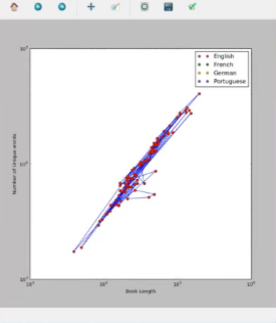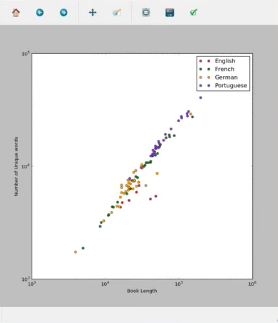# Text Analysis in Python 3

Book’s / Document’s Content Analysis

Patterns within written text are not the same across all authors or languages.This allows linguists to study the language of origin or potential authorship of texts where these characteristics are not directly known such as the Federalist Papers of the American Revolution.

Aim: In this case study, we will examine the properties of individual books in a book collection from various authors and various languages.More specifically, we will look at book lengths, number of unique words, and how these attributes cluster by language of or authorship.

Source: Project Gutenberg is the oldest digital library of books.It aims to digitize and archive cultural works, and at present, contains over 50, 000 books, all previously published and now available electronically.Download some of these English & French books from here and the Portugese & German books from here for analysis.Put all these books together in a folder called Books with subfolders English, French, German & Potugese.

Word Frequency in Text

So we are going to build a function which will count the word frequency in a text.We will consider a sample test text, & later will replace the sample text with the text file of books that we have just downloaded.Since we are going to count word frequency, therefore UPPERCASE and lowercase letters are the same.We will convert the whole text into lowercase and save it.

 `text ``=` `"This is my test text. We're keeping this text short to keep things manageable."` `text ``=` `text.lower() `

Word frequency can be counted in various ways.We are going to code, two such ways ( just for knowledge ).One using for loop and the other using Counter from collections, which proves to be faster than the previous one.The function will return a dictionary of unique words & its frequency as a key-value pair.So, we code:

 `from` `collections ``import` `Counter  ` ` `  `# counts word frequency ` `def` `count_words(text):                   ` `    ``skips ``=` `[``"."``, ``", "``, ``":"``, ``";"``, ``"'", '"']  ` `    ``for ch in skips:  ` `        ``text = text.replace(ch, "")  ` `    ``word_counts = {}  ` `    ``for word in text.split(" "):  ` `        ``if word in word_counts:  ` `            ``word_counts[word]+= 1  ` `        ``else:  ` `            ``word_counts[word]= 1  ` `    ``return word_counts  ` ` `  `    ``# >>>count_words(text) You can check the function  ` ` `  `# counts word frequency using ` `# Counter from collections  ` `def count_words_fast(text):      ` `    ``text = text.lower()  ` `    ``skips = [".", ", ", ":", ";", "'"``, '"']  ` `    ``for` `ch ``in` `skips:  ` `        ``text ``=` `text.replace(ch, "")  ` `    ``word_counts ``=` `Counter(text.split(``" "``))  ` `    ``return` `word_counts  ` ` `  `    ``# >>>count_words_fast(text) You can check the function  `

Output : The output is a dictionary holding the unique words of the sample text as key and the frequency of each word as value.Comparing the output of both the functions, we have:

{‘were’: 1, ‘is’: 1, ‘manageable’: 1, ‘to’: 1, ‘things’: 1, ‘keeping’: 1, ‘my’: 1, ‘test’: 1, ‘text’: 2, ‘keep’: 1, ‘short’: 1, ‘this’: 2}

Counter({‘text’: 2, ‘this’: 2, ‘were’: 1, ‘is’: 1, ‘manageable’: 1, ‘to’: 1, ‘things’: 1, ‘keeping’: 1, ‘my’: 1, ‘test’: 1, ‘keep’: 1, ‘short’: 1})

Reading Books into Python: Since, we were successful in testing our word frequency functions with the sample text.Now, we are going to text the functions with the books, which we downloaded as text file.We are going to create a function called read_book() which will read our books in Python and save it as a long string in a variable and return it.The parameter to the function will be the location of the book.txt to be read and will be passed while calling the function.

 `#read a book and return it as a string ` `def` `read_book(title_path):   ` `    ``with ``open``(title_path, ``"r"``, encoding ``=``"utf8"``) as current_file: ` `        ``text ``=` `current_file.read() ` `        ``text ``=` `text.replace(``"\n"``, "``").replace("``\r``", "``") ` `    ``return` `text `

Total Unique words: We are going to design another function called word_stats(), which will take the word frequency dictionary( output of count_words_fast()/count_words() ) as a parameter.The function will return the total no of unique words(sum/total keys in the word frequency dictionary) and a dict_values holding total count of them together, as a tuple.

 `# word_counts = count_words_fast(text) ` `def` `word_stats(word_counts):       ` `    ``num_unique ``=` `len``(word_counts)  ` `    ``counts ``=` `word_counts.values()  ` `    ``return` `(num_unique, counts)  `

Calling the functions: So, lastly we are going to read a book, for instance – English version of Romeo and Juliet, and collect information on word frequency, unique words, total count of unique words etc from the functions.

 `text ``=` `read_book(``"./Books / English / shakespeare / Romeo and Juliet.txt"``) ` ` `  `word_counts ``=` `count_words_fast(text)          ` `(num_unique, counts) ``=` `word_stats(word_counts) ` `print``(num_unique, ``sum``(counts))  `

```Output: 5118 40776
```

With the help of the functions that we created, we came to know that there are 5118 unique words in the English version of Romeo and Juliet and the Sum of frequency of the unique words sums up to 40776.We can know which word occurred the most in the book & can play with different versions of books, of different languages to know about them and their stats with the help of above functions.

Plotting Characteristic Features of Books

We are going to plot, (i)Book length Vs Number of Unique words for all the books of different languages using matplotlib.We will import pandas to create a pandas dataframe, which will hold information on books as columns.We will categorize these columns by different categories such as – “language”, “author”, “title”, “length” & “unique” .To plot book length along x axis and Number of unique words along y axis, we code:

 `import` `os  ` `import` `pandas as pd  ` ` `  `book_dir ``=` `"./Books"` `os.listdir(book_dir)  ` ` `  `stats ``=` `pd.DataFrame(columns ``=``(``"language"``, ` `                               ``"author"``, ` `                               ``"title"``, ` `                               ``"length"``, ` `                               ``"unique"``))  ` ` `  `# check >>>stats  ` `title_num ``=` `1` `for` `language ``in` `os.listdir(book_dir):  ` `    ``for` `author ``in` `os.listdir(book_dir``+``"/"``+``language):  ` `        ``for` `title ``in` `os.listdir(book_dir``+``"/"``+``language``+``"/"``+``author): ` `             `  `            ``inputfile ``=` `book_dir``+``"/"``+``language``+``"/"``+``author``+``"/"``+``title  ` `            ``print``(inputfile)  ` `            ``text ``=` `read_book(inputfile)  ` `            ``(num_unique, counts) ``=` `word_stats(count_words_fast(text))  ` `            ``stats.loc[title_num]``=` `language, ` `            ``author.capitalize(), ` `            ``title.replace(``".txt"``, ""),  ` `            ``sum``(counts), num_unique  ` `            ``title_num``+``=` `1` `import` `matplotlib.pyplot as plt  ` `plt.plot(stats.length, stats.unique, ``"bo-"``)  ` ` `  `plt.loglog(stats.length, stats.unique, ``"ro"``)  ` ` `  `stats[stats.language ``=``=``"English"``] ``#to check information on english books  ` `     `  `plt.figure(figsize ``=``(``10``, ``10``))  ` `subset ``=` `stats[stats.language ``=``=``"English"``]  ` `plt.loglog(subset.length, ` `           ``subset.unique, ` `           ``"o"``, ` `           ``label ``=``"English"``, ` `           ``color ``=``"crimson"``)  ` ` `  `subset ``=` `stats[stats.language ``=``=``"French"``]  ` `plt.loglog(subset.length, ` `           ``subset.unique, ` `           ``"o"``, ` `           ``label ``=``"French"``, ` `           ``color ``=``"forestgreen"``)  ` ` `  `subset ``=` `stats[stats.language ``=``=``"German"``]  ` `plt.loglog(subset.length, ` `           ``subset.unique, ` `           ``"o"``, ` `           ``label ``=``"German"``, ` `           ``color ``=``"orange"``)  ` ` `  `subset ``=` `stats[stats.language ``=``=``"Portuguese"``]  ` `plt.loglog(subset.length, ` `           ``subset.unique, ` `           ``"o"``, ` `           ``label ``=``"Portuguese"``, ` `           ``color ``=``"blueviolet"``)  ` ` `  `plt.legend()  ` `plt.xlabel(``"Book Length"``)  ` `plt.ylabel(``"Number of Unique words"``)  ` `plt.savefig(``"fig.pdf"``)  ` `plt.show()  `

Output: We plotted two graphs, the first one representing every book of different language & author as simply a book.The red dots in the first graph represent a single book and they are connected by blue lines.The loglog plot creates discrete points [red here] and the linear plot creates linear curves [blue here], joining the points.The second graph is a logarithmic plot which displays books of different languages with different colours [red for English, Green for French etc] as discrete points.
These graphs help in analysis facts visually about different books of vivid origin.From the graph, we came to know that Portugese books are longer in length and have greater number of unique words than German or English books.Plotting such data proves to be of great help for linguists.Reference :

This article is contributed by Amartya Ranjan Saikia. If you like GeeksforGeeks and would like to contribute, you can also write an article using contribute.geeksforgeeks.org or mail your article to contribute@geeksforgeeks.org. See your article appearing on the GeeksforGeeks main page and help other Geeks.

Attention geek! Strengthen your foundations with the Python Programming Foundation Course and learn the basics.

To begin with, your interview preparations Enhance your Data Structures concepts with the Python DS Course.

My Personal Notes arrow_drop_up
Article Tags :

2

Please write to us at contribute@geeksforgeeks.org to report any issue with the above content.